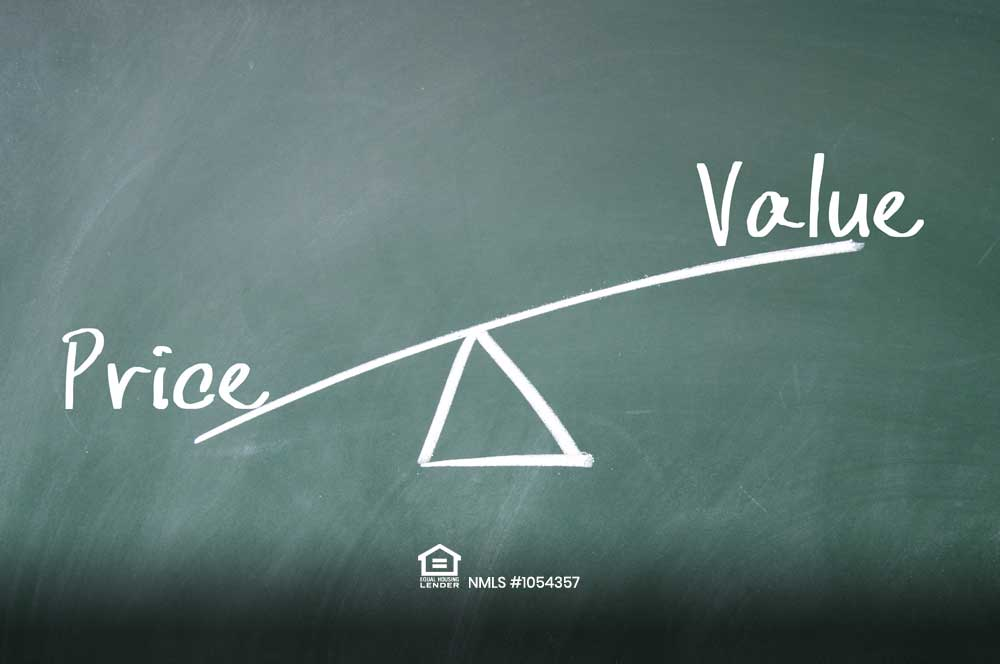#### What is a Loan to Value?This is the percentage that compares the loan to the value (LTV) of the property. For example, if you are putting down 20% on a property, the LTV is 80%. A LTV ratio is a term used by lenders to express the ratio of a loan to the value of an asset purchased. The LTV ratio is calculated by the amount of the mortgage lien divided by the appraised value of a property. The higher your LTV ratio is, the lower your home equity. Having less than 80% of a loan to value presents less risk to lenders. A LTV ratio can affect interest rates. If the LTV ratio is 75% or lower, the borrower may see a decrease in interest rates.

The mortgage rate you will get is affected by the LTV thresholds, the lower it is the cheaper the rate. If you have a 90 percent loan to value. This could mean as if you put in 10 percent on a home, you are financing 90 percent of the equity of the home. A good LTV percentage is at 80%.